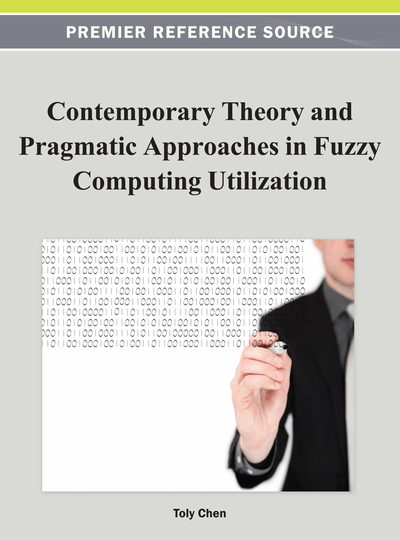# A New Efficient and Effective Fuzzy Modeling Method for Binary Classification

T. Warren Liao (Louisiana State University, USA)
DOI: 10.4018/978-1-4666-1870-1.ch004
Available
\$37.50
No Current Special Offers

## Abstract

This paper presents a new fuzzy modeling method that can be classified as a grid partitioning method, in which the domain space is partitioned by the fuzzy equalization method one dimension at a time, followed by the computation of rule weights according to the max-min composition. Five datasets were selected for testing. Among them, three datasets are high-dimensional; for these datasets only selected features are used to control the model size. An enumerative method is used to determine the best combination of fuzzy terms for each variable. The performance of each fuzzy model is evaluated in terms of average test error, average false positive, average false negative, training error, and CPU time taken to build model. The results indicate that this method is best, because it produces the lowest average test errors and take less time to build fuzzy models. The average test errors vary greatly with model sizes. Generally large models produce lower test errors than small models regardless of the fuzzy modeling method used. However, the relationship is not monotonic. Therefore, effort must be made to determine which model is the best for a given dataset and a chosen fuzzy modeling method.
Chapter Preview
Top

## Introduction

Binary classification refers to the task of classifying an unknown object into one of the two known classes. Many real world problems can be formulated as binary classification problems. The examples are numerous, which include determining whether a patient has certain disease or not, determining whether an artifact is defective or not, determining whether an email is a spam or not, and so on.

Before classification can be carried out, a classification system or a classifier must be built first. A classification system or classifier built for performing binary classification is called a binary classifier. Many machine learning methods such as decision trees, neural networks, support vector machines, and fuzzy models are suitable for building binary classifiers. Of interest in this paper are fuzzy models.

A fuzzy model is comprised of a set of fuzzy if-then rules. Various forms of fuzzy rules are possible. Most well-known among them are Mamdani linguistic rules and TSK rules, as described below for an n-input and single-output system.

• Mamdani linguistic rules are of the form of Ri: If X1 is A1i and X2 is A2i, …, and Xn is Ani, then Y is Bi. In each Mamdani rule, Xn denotes the nth input variable, Ani the fuzzy set associated with An, and Bi the fuzzy set associated with output variable Y of rule Ri. Bi can be represented by its modal value or as a fuzzy singleton for fuzzy control and classification applications.

• TSK rules are of the form of Ri: If X1 is A1i and X2 is A2i, …, and Xn is Ani, then Y = b0i + b1iX1 + b2iX2 + … + bniXn. In each TSK rule, Xn denotes the nth input variable, Ani the fuzzy set associated with An, and bi is a parameter vector and b0i is a scalar offset associated with rule Ri. If all parameters in the vector bi are zeros, then TSK rules are identical to Mamdani rules with Bi being fuzzy singletons.

A fuzzy model built for classification consists of a set of fuzzy classification rules of the following form Ri: If X1 is A1i and X2 is A2i, …, and Xn is Ani, then Y is Bi. In each classification rule, Xn denotes the nth input variable, Ani the fuzzy set associated with An, and Bi ∈ {B1, B2, …, Bm} representing the class label of rule Ri. For binary classification problems, there are only two values, i.e., Bi ∈ {B1, B2}. Fuzzy classification rules are identical to Mamdani rules with Bi being fuzzy singletons or TSK rules with bi being zeros. Each rule could be assigned a rule weight. If not explicitly assigned, each rule is considered having rule weight of one.

Fuzzy models can be built manually or interactively using a tool designed to do that, e.g. the FIS Editor in the Matlab fuzzy toolbox. These approaches, however, require a priori knowledge about the model. The alternative is to build fuzzy models from data for which numerous methods have been proposed. These data-driven methods can be grouped into the following categories (Liao, 2006):

## Complete Chapter List

Search this Book:
Reset